# How to use Excel COUNTIFS and COUNTIF with multiple criteria

The tutorial explains how to use COUNTIFS and COUNTIF formulas with multiple criteria in Excel based on AND as well as OR logic. You will find a number of examples for different data types - numbers, dates, text, wildcard characters, non-blank cells and more.

Of all Excel functions, COUNTIFS and COUNTIF are probably most often mixed up because they look very much alike and both are purposed for counting cells based on the specified criteria.

The difference is that COUNTIF is designed for counting cells with a single condition in one range, whereas COUNTIFS can evaluate different criteria in the same or in different ranges. The aim of this tutorial is to demonstrate different approaches and help you choose the most efficient formula for each particular task.

## Excel COUNTIFS function - syntax and usage

As already mentioned, the Excel COUNTIFS function is purposed for counting cells across multiple ranges based on one or several conditions. The COUNTIFS function is available in Excel 2016, 2013, Excel 2010, and Excel 2007, so you can use the below examples in any Excel version.

### COUNTIFS syntax

The syntax of the COUNTIFS function is as follows:

COUNTIFS(criteria_range1, criteria1, [criteria_range2, criteria2]…)
• criteria_range1 - defines the first range to which the first condition (criteria1) shall be applied, required.
• criteria1 - sets the condition in the form of a numbercell referencetext stringexpression or another Excel function, required. The criteria defines which cells shall be counted and can be expressed as 10, "<=32", A6, "sweets".
• [criteria_range2, criteria2]… - these are additional ranges and their associated criteria, optional. You can specify up to 127 range/criteria pairs in your formulas.

In fact, you don't have to remember the syntax of the COUNTIF function by heart. Microsoft Excel will display the function's arguments as soon as you start typing; the argument you are entering at the moment is highlighted in bold.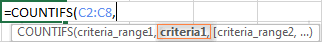### Excel COUNTIFS - things to remember!

1. You can use the COUNTIFS function in Excel to count cells in a single range with a single condition as well as in multiple ranges with multiple conditions. If the latter, only those cells that meet all of the specified conditions are counted.
2. Each additional range must have the same number of rows and columns as the first range (criteria_range1 argument).
3. Both contiguous and non-contiguous ranges are allowed.
4. If the criteria is a reference to an empty cell, the COUNTIFS function treats it as a zero value (0).
5. You can use the wildcard characters in criteria - asterisk (*) and question mark (?). See this example for full details.

## How to use COUNTIFS and COUNTIF with multiple criteria in Excel

Below you will find a number of formula examples that demonstrate how to use the COUNTIFS and COUNTIF functions in Excel to evaluate multiple conditions.

### How to count cells with multiple criteria (AND logic)

This scenario is the easiest one, since the COUNTIFS function in Excel is designed to count only those cells for which all of the specified conditions are TRUE. We call it the AND logic, because Excel's AND function works this way.

#### Formula 1. COUNTIFS formula with multiple criteria

Suppose you have a product list like shown in the screenshot below. You want to get a count of items that are in stock (value in column B is greater than 0) but have not been sold yet (value is column C is equal to 0).

The task can be accomplished by using this formula:

`=COUNTIFS(B2:B7,">0", C2:C7,"=0")`

And the count is 2 ("Cherries" and "Lemons"):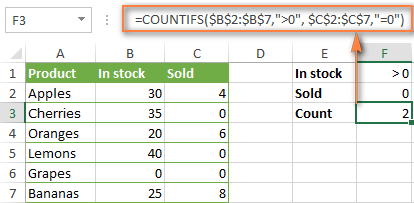#### Formula 2. COUNTIFS formula with the same criteria

When you want to count items with identical criteria, you still need to supply each criteria_range / criteria pair individually.

For example, here's the right formula to count items that have 0 both in column B and column C:

`=COUNTIFS(\$B\$2:\$B\$7,"=0", \$C\$2:\$C\$7,"=0")`

This COUNTIFS formula returns 1 because only "Grapes" have "0" value in both columns.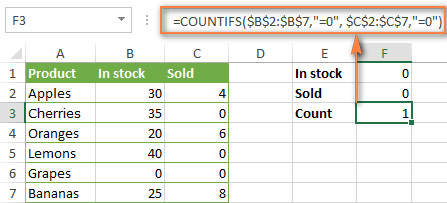Using a simpler formula with a single criteria_range like COUNTIFS(B2:C7,"=0") would yield a different result - the total count of cells in the range B2:C7 containing a zero (which is 4 in this example).

### How to count cells with multiple criteria (OR logic)

As you have seen in the above examples, counting cells that meet all of the specified criteria is easy because the COUNTIFS function is designed to work this way.

But what if you want to count cells for which at least one of the specified conditions is TRUE, i.e. based on the OR logic? Overall, there are two ways to do this - by adding up several COUNTIF formulas or using a SUM COUNTIFS formula with an array constant.

#### Formula 1. Add up two or more COUNTIF or COUNITFS formulas

In the table below, supposing you want to count orders with the "Cancelled" and "Pending" status. To have it doen, you can simply write 2 regular Countif formulas and add up the results:

`=COUNTIF(\$C\$2:\$C\$11,"Cancelled") + COUNTIF(\$C\$2:\$C\$11,"Pending")`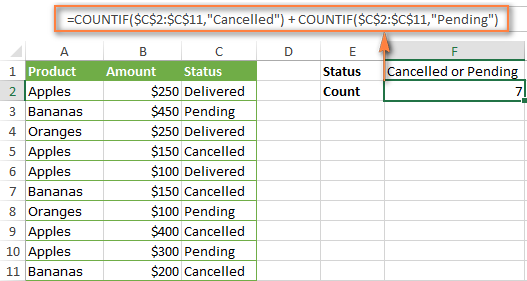In case each of the functions is supposed to evaluate more than one condition, use COUNTIFS instead of COUNTIF. For example, to get the count of "Cancelled" and "Pending" orders for "Apples" use this formula:

`=COUNTIFS(\$A\$2:\$A\$11, "Apples", \$C\$2:\$C\$11,"Cancelled") + COUNTIFS(\$A\$2:\$A\$11, "Apples", \$C\$2:\$C\$11,"Pending")`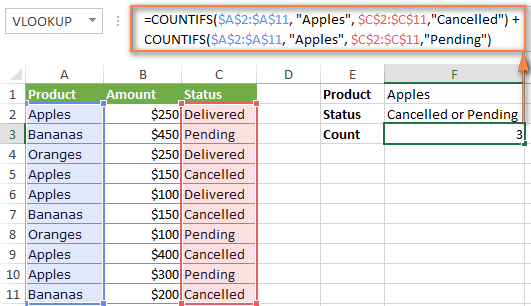#### Formula 2. SUM COUNTIFS with an array constant

In situations when you have to evaluate a lot of criteria, the above approach is not the best way to go because your formula would grow too big in size. To perform the same calculations in a more compact formula, list all of your criteria in an array constant, and supply that array to the criteria argument of the COUNTIFS function. To get the total count, embed COUNTIFS inside the SUM function, like this:

SUM(COUNTIFS(range,{"criteria1","criteria2","criteria3",…}))

In our sample table, to count orders with the status "Cancelled" or "Pending" or "In transit", the formula would go as follows:

`=SUM(COUNTIFS(\$C\$2:\$C\$11, {"cancelled", "pending", "in transit"}))`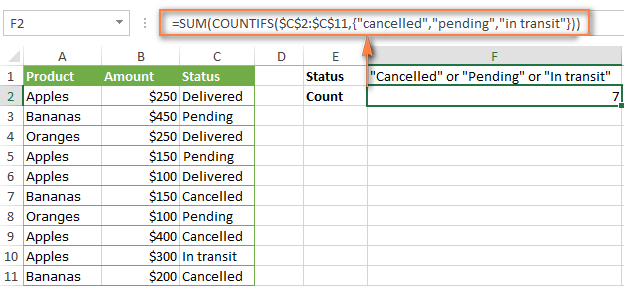In a similar manner, you can count cells based on two or more criteria_range / criteria pairs. For instance, to get the number of "Apples" orders that are "Cancelled" or "Pending" or "In transit", use this formula:

`=SUM(COUNTIFS(\$A\$2:\$A\$11,"apples",\$C\$2:\$C\$11,{"cancelled","pending","in transit"}))`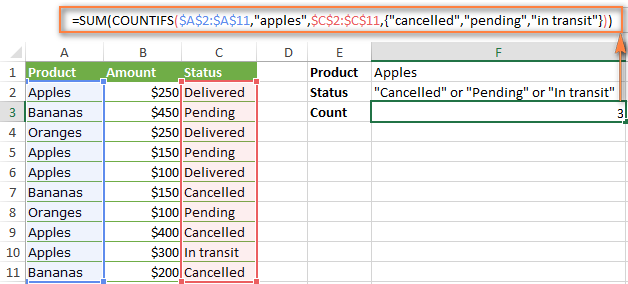You can find a few more ways to count cells with OR logic in this tutorial: Excel COUNTIF and COUNTIFS with OR conditions.

### How to count numbers between 2 specified numbers

By and large, COUNTIFS formulas for numbers fall into 2 categories - based on several conditions (explained in the above examples) and between the two values you specify. The latter can be accomplished in two ways - by using the COUNTIFS function or by subtracting one COUNTIF from another.

#### Formula 1. COUNTIFS to count cells between two numbers

To find out how many numbers between 5 and 10 (not including 5 and 10) are contained in cells C2 through C10, use this formula:

=COUNTIFS(C2:C10,">5", C2:C10,"<10")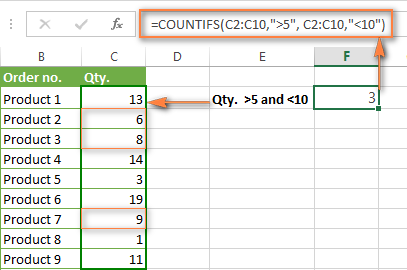To include 5 and 10 in the count, use the "greater than or equal to" and "less than or equal to" operators:

=COUNTIFS(B2:B10,">=5", B2:B10,"<=10")

#### Formula 2. COUNTIF formulas to count numbers between X and Y

The same result can be achieved by subtracting one Countif formula from another. The first one counts how many numbers are greater than the lower bound value (5 in this example). The second formula returns the count of numbers that are greater than the upper bound value (10 in this case). The difference between the first and second number is the result you are looking for.

• =COUNTIF(C2:C10,">5")-COUNTIF(C2:C10,">=10") - counts how many numbers greater than 5 and less than 10 are in the range C2:C10. This formula will return the same count as shown in the screenshot above.
• =COUNTIF(C2:C10, ">=5")-COUNTIF(C2:C10, ">10") - the formula counts how many numbers between 5 and 10 are in the range C2:C10, including 5 and 10.

### How to use cell references in COUNTIFS formulas

When using logical operators such as ">", "<", "<=" or ">=" together with cell references in your Excel COUNTIFS formulas, remember to enclose the operator in "double quotes" and
add an ampersand (&) before a cell reference to construct a text string.

In a sample dataset below, let's count "Apples" orders with amount greater than \$200. With criteria_range1 in cells A2:A11 and criteria_range2 in B2:B11, you can use this formula:

`=COUNTIFS(\$A\$2:\$A\$11, "Apples", \$B\$2:\$B\$11, ">200")`

Or, you can input your criteria values in certain cells, say F1 and F2, and reference those cells in your formula:

`=COUNTIFS(\$A\$2:\$A\$11, \$F\$1, \$B\$2:\$B\$11, ">"&\$F\$2)`

Please notice the use of absolute cell references both in the criteria and criteria_range arguments, which prevents the formula from being broken when copied to other cells.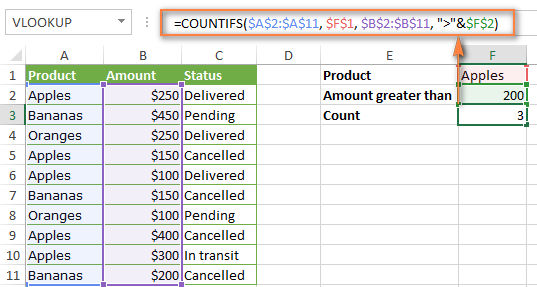For more information about the use of an ampersand in COUNTIF and COUNTIFS formulas, please see Excel COUNTIF - frequently asked questions.

### How to use COUNTIFS with wildcard characters

In Excel COUNTIFS formulas, you can use the following wildcard characters:

• Question mark (?) - matches any single character, use it to count cells starting and/or ending with certain characters.
• Asterisk (*) - matches any sequence of characters, you use it to count cells containing a specified word or a character(s) as part of the cell's contents.
Tip. If you want to count cells with an actual question mark or asterisk, type a tilde (~) before an asterisk or question mark.

Now let's see how you can use a wildcard char in real-life COUNTIFS formulas in Excel. Suppose, you have a list of projects in column A. You wish to know how many projects are already assigned to someone, i.e. have any name in column B. And because we are learning how to use the COUNTIFS function with multiple criteria, let's add a second condition - the End Date in column D should also be set.

Here is the formula that works a treat:

=COUNTIFS(B2:B10,"*",D2:D10,"<>"&""))

Please note, you cannot use a wildcard character in the 2nd criteria because you have dates rather that text values in column D. That is why, you use the criteria that finds non-blank cells: "<>"&""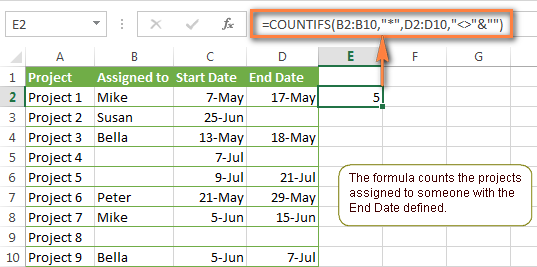### COUNTIFS and COUNTIF with multiple criteria for dates

The COUNTIFS and COUNTIF formulas you use for dates are very much similar to the above formulas for numbers.

#### Example 1. Count dates in a specific date range

To count the dates that fall in a certain date range, you can also use either a COUNTIFS formula with two criteria or a combination of two COUNTIF functions.

For example, the following formulas count the number of dates in cells C2 through C10 that fall between 1-Jun-2014 and 7-Jun-2014, inclusive:

`=COUNTIFS(C2:C9, ">=6/1/2014", C2:C9, "<=6/7/2014")`

`=COUNTIF(C2:C9, ">=6/1/2014") - COUNTIF(C2:C9, ">6/7/2014")`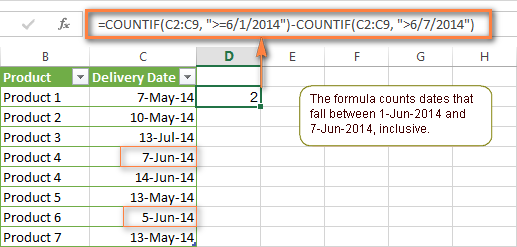#### Example 2. Count dates with multiple conditions

In the same manner, you can use a COUNTIFS formula to count the number of dates in different columns that meet 2 or more conditions. For instance, the below formula will find out how many products were purchased after the 20th of May and delivered after the 1st of June:

`=COUNTIFS(C2:C9, ">5/1/2014", D2:D9, ">6/7/2014")`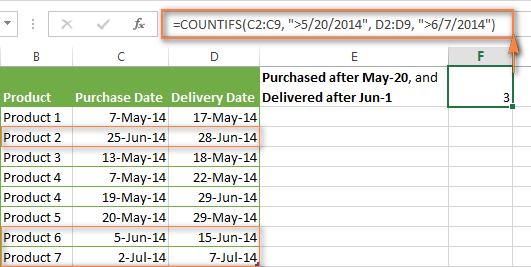#### Example 3. Count dates with multiple conditions based on the current date

You can use Excel's TODAY() function in combination with COUNTIF to count dates based on the current date.

For example, the following COUNTIF formula with two ranges and two criteria will tell you how many products have already been purchased but not delivered yet.

`=COUNTIFS(C2:C9, "<"&TODAY(), D2:D9, ">"&TODAY())`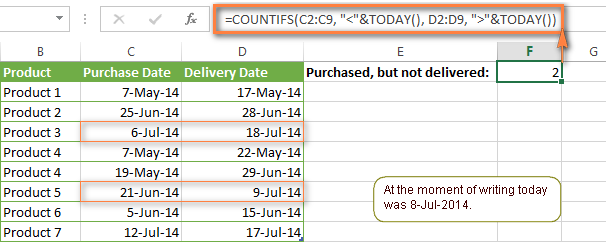This formula allows for many possible variations. For instance, you can tweak it to count how many products were purchased more than a week ago and are not delivered yet:

`=COUNTIFS(C2:C9, "<="&TODAY()-7, D2:D9, ">"&TODAY())`

This is how you count cells with multiple criteria in Excel. I hope you will find these examples helpful. Anyway, I thank you for reading and hope to see you on our blog next week!

### 1,045 Responses to "How to use Excel COUNTIFS and COUNTIF with multiple criteria"

1.JVal says:

Good Afternoon,
I have created a checklist in Excel with checkboxes under each heading (3 headings) with the option of TRUE,FALSE or #N/A (as some of the items may not be applicable). I have assigned a cell to each check box. I am trying to capture the completion rate of the items required in my checklist (both when required and/or applicable information has been received). I have used COUNTIF to capture the TRUE data in each subheading, but I cant seem to figure out a formula to capture the required data and additional data when applicable to score as available points and actual points. Any ideas?

2.Roberto Avila says:

I am searching on how to count cells based on specific text within a date range.

E.G. I have a column with codes: UA, RO, etc. I have another column with the date the the code was created.

I need to count the number of cells with UA from 09-01-2018 and 09-30-2018.

I find the syntax for counting a cell with the text "code" and how to "sum" numeric values in a date range. I just don't seem to be able to find the syntax that combines both counting cells for specific text within a date range.

Anybody know how?

3.PARTHA SARATHI BISWAS says:

how i will calculate the total number of attendance,like Absent and Present in a month? my excel work sheet is given below:-

(A=Absent,P=Present)

01-Nov
SE Name Remarks
Chan A

02-Nov
SE Name Remarks
Chan P

03-Nov
SE Name Remarks
Chan P

Pls.help me...

Thanks.
P.Biswas.

4.Doug says:

Partha:
You can count multiple criteria by using the COUNTIFS function.
In your case where the first column is in A67:A81 and the attendance is in B67:B81 put "Mad" in C69, "Dad" in C70 and "Chad" in C71. Then in D69 enter =COUNTIFS(A67:A81,C69,B67:B81,"P")and in D70 enter =COUNTIFS(A67:A81,C70,B67:B81,"P")and in D71 enter =COUNTIFS(A67:A81,C71,B67:B81,"P").
You'll have the attendance for the Nov range next to each name.

5.Mandy says:

I am trying to figure out a formula that if P2 is =1000 add 120 days to the date in M2. I cannot seem to get it to return a correct answer. Any help is greatly appreciated.

•Mandy says:

I should clarify that cell M2 is formatted as a date.

=Countifs(M2,P2,">=1000",M2,120,P2,"<999",M2,90)
I'm sure my formula is no where close to where it needs to be.

6.Mohammed Ali says:

Dear,

Please help me with the table. I would like to change the data when I change the Month. It should change only the data from the table. Month is in dropdown list.

October ACC AOP ASC
DS 98.91% 93.33% 93.18%
KD 100.00% 75.50% 88%
HP 86.91% 89.13% 88%

Regards,
MZA

7.John Nelson says:

In each row (twenty schools) I have two columns (B, C) of attendees (student, non-nonstudent). How would I count whether a school is represented by either a student or a non-student (i.e., count if b=>0 or if c=>0)?

I tried =countifs (B3:B22,">0", C3:C22,">0") and tried =countifs (B3:B22,">0") + countifs (C3:C22,">0"). Neither of these worked.

•Mohammed Ali says:

Could you please send me the table, so that I can do it.

8.Jaisankar says:

Dear friends, help to simply my work in excel. I have daily stocks data in A1:A100 for day 1 and B1:B100 for day 2. Now i want to compare B column with A and result to display in C as how many times increased or decreased. i.e 2X times increased, 5X times decreased like.

Is there anyway to setup formula for my requirements...

9.Tghanem says:

I have huge excel where the data duplicated. The frame of the table has:
1- Student name "Duplicated"
2- Class "Duplicated"
3- Subject "Duplicated"
4- Exam as Pass,Fail.Pending. "Duplicated"
5- Date "Duplicated"
6- Record of data entry is IDENTICAL.
The project's owner wants to know the percentage of the student pass the exam(per class & subject) in a table where the row is the class and the column is subject. The issue here as the student had repeated the exam different times in both different classes and subjects. The owner is accepting to have one exam result within a three month period if the student repeated the same exam in the same class and for same subject.
I used countifs but its really exhausting exercise for huge data. Is there any other solution ?!!

10.Tghanem says:

The project's owner wants to know the percentage of the student pass the exam(per class & subject) in a table where the row is the class and the column is subject. The issue here as the student had repeated the exam different times in both different classes and subjects. The owner is accepting to have one exam result within a three month period if the student repeated the same exam in the same class and for same subject.
I used countifs but its really exhausting exercise for huge data. Is there any other solution ?!!

11.maqbul says:

good evening
kindly help me out

i have a excel table included with departments and dates

i have 4 types of departments and dates from jan to dec
i need to combine the BELOW GIVEN BOTH forumla IN ONE like example given below

=COUNTIFS(A2:A7,"Logistics Department")
other for formula is

=COUNTIFS(B2:B7, "="01/01/2018)

MY MAIL ID maqbul2005@gmail.com

•Mary Trifuntova (Ablebits.com Team) says:

Hello, Maqbul,
Please try to apply the formula below:

=COUNTIFS(A2:A7,"Logistics Department", B2:B7, "="&DATE(2018,1,1))

You can learn more about COUNTIFS with multiple criteria in Excel in this article on our blog.
Hope you’ll find this information helpful.

12.Gary says:

looking for some advice, I'm trying to count different values in excel or sheets.

example: counting sick days for employees.

Full sick day being 1
half sick day being 0.5

SICK = 1
SICK/ = 0.5 (this means the first half of the day was sick)
/SICK = 0.5 (this means the second half of the day was sick)

How can I build something that recognizes SICK SICK/ and /SICK and also adds up the different values of each. Thanks in advance.

13.robin says:

I am trying to create a formula where I am counting only answers that are NA or Pass. I am able to successfully create the formula if i am just counting Pass, but not both. I am not using a range. The formula that works for just pass is: =SUM((COUNTIF(INDIRECT({"B10","B13","B17","B18"}),"Pass")*D10),"0")

How do I also make it add NA?

14.Aufa Fadhli says:

Hi,

Kindly help me, I have some criteria to be defined from below table:
Master Try 1 Try 2
G G R
G G R
G G G
R R R
R G G

From above you see G (good) and R (reject). What I what to count is correctly decision and falsely decision referring to master. The table will like below:
CORRECTLY FALSELY
G R G R

Aufa

15.shazza says:

Hi ,

I wanted to countif there is "3" and there is differences in Row 1 , than it will count , I should have the answer of 2
( Under Apple and pear there is "3" )
How should I compute the formula .
P/S : I want to count Row B "3" if there is Unique data in Row A.

Apple Orange Pear Apple Apple Orange
3 3 3

16.Mohan says:

I have a question with Countifs..

Can i have the criteria rage more than 1 column ?

eg: I have 3 columns which has "Critical, High & Medium" and one column with countries (India , Pakistan).

Now i need to know how many critical in India.

17.debasish roy says:

how do we split during COUNT when A1=bc
we want b1 shows 1 for A1 =bc & c1 shows 1 for A1=bc

18.Eyale says:

Hi there,

Am I able to use the ABS function on this formula somehow?

=SUM(COUNTIFS('Sheet1'!A\$2:A\$191,{"0*","1*","2*","3*","4*","5*","6*","7*","8*","9*"},'Sheet1'!E\$2:E\$191,">"&F8))

I tried the following but it is not working:

=SUM(COUNTIFS('Sheet1'!A\$2:A\$191,{"0*","1*","2*","3*","4*","5*","6*","7*","8*","9*"},ABS('Sheet1'!E\$2:E\$191),">"&F8))

Is there something I am missing here? Tried all sorts of strategies around it and I am not able to do it. Please help.

19.Raffy says:

Hi, Can you help me with this?

I want to get the numbers of "banned" items on a specific date? if my list is included with deleted items.

20.Cora Blew says:

I cant figure out my error =COUNTIFS(G2:G75,"PAC", Q2:QR75,"Pending", COUNT L2:L75,"Case Manager")

21.Jason says:

When I use Countif, I highlight the range which enters correctly (C2:C295, but when I click the first cell that I want to count for the criteria it just enters "Extract" in the field and doesn't work. I've done this before and always have issues but eventually figure it out. Why doesn't it just read the value when I click the cell? Why does it say Extract and then not count how many times the value appears in the range????

•Jason says:

I copied the criteria column and pasted as values into another column since apparently excel can't see the values as they are so now not getting extract when I select the criteria. However, when I hit enter - the cell just displays the formula rather than the results!!! So my cell says =COUNTIF(C2:C295,E2) instead of "4" or whatever the count would be. wth am I doing wrong??????????????O

22.VISHAL says:

IF SUPPOSE CELL HAVING 31-JAN-2020 IS DUE DATE, BUT BEFORE 300 THE SAME CELL NEEDS REFLECT IN RED COLOR

23.VISHAL says:

AMC End Date
31/01/2020
How to put conditional format for above date before 30 day's it should reflect in red color means it's come for due.
Kindy help with countif conditional formulas

24.Asad says:

Is there any way to count non zero cells when cells to be checked are not in a series. For example, I want to count non zero cells from these 5 cells A1, B23, C21, A11,D13

25.tammy says:

I are in need of some help. I know a little bit of excel but not what my boss needs. Column C has the dates that items will be shipped, column d has if the item will be lined, column e has if the item will be blasted, and column f has if the item will be painted. She is wanting me to have a formula calculate the items for each column on a certain date, if that makes sense. For example: On day 04/12/2019, we want to know how many items will be shipped that were lined, blasted or painted separately. for each cell, there is the dollar amount.

26.Christina says:

Hello,
I was wondering if you could help me please - I need to create a formula that counts the number of times there is an overdue date in column I, where the corresponding cell in column J is blank.
A date is entered into column H (date appointed), there is a formula to auto-fill column I (date due) which is =IF(ISBLANK(H6), " ", EDATE(H6,6))
There is also conditional formatting on column I which turns the date red if it meets this criteria. =AND(\$I6<TODAY(), \$J6="")
An entry into column J (date reviewed) results in the conditional formatting being removed, so that it returns to black.
I need to create a formula to count the number of dates in column I which are older than today but only if the corresponding cell in column J is blank.
I have tried =COUNTIFS(I6:I260,"<TODAY()",J6:J260,""&"""") but suspect it is counting the auto-fill formula in column I. Please help!!!!
thanks
-C

27.tami says:

I have a column for genders and one for diferent races
I need to use excel to calculate number of males and also females for each race separating the males and females

28.A says:

In your Product, Amount, Status data range, how would you find the Number of Fruits Canceled?

So in this example, the goal would be to get a unique count of Product that was Canceled. Bananas and Apples show up twice, but the result would only return the unique instances of Canceled for each. Thank you!

29.Dion says:

I need a formula that finds the words "Email Sales" in column A:A and adds the amounts that correspond from column B:B. In the below example, my result should be \$32,000

Email Sales \$15,000
Email Sales \$2,200
Email Sales \$2,800
Email Sales \$12,000

30.cph says:

I have a requirement which involves countif. I have a sequence of Tif files
0557_0001 - sequence 2
0557_0003 - Sequence 4
0557_0007 - Sequence 3
The file no 0557_0001 has 2 pages which is refereed as sequence ie is Sequence 2.
When we add : file no 0557_0001 + 2 pages gives 0557_0003
I have used =COUNTIFS(B:B,B1) to get the sequence.I need to include the addition part and populate which does not tally the sequence.
Example: When we add : file no 0557_0001 + 2 pages gives 0557_0003 but if i have the next file as 0557_0004 , it should populate the difference as -1.

31.ARPIT GUPTA says:

Hi
I need to know which formula is put in condtion :-
if someone do 12 hrs duty in a day but we take 8hr per day & 4 hrs as an ot(over time) payment should be make by diff rates of 8hr & 4rs ot.

32.JASON UCHE says:

I have created four sheets in Excel to record monthly attendance in church. The first sheet records the Guest that are attending for the first time(first time guest list), the second sheet Records guests that attended for the second time (i.e same guest attending again). The third sheet is for analysis where I plan to display the number of total First time guests showing how many are male and female). My problem is I have done the total guest per month it works well, but i am not able to extract the number of Male and female from the (first time guest list). Sheet1(Month, Name, Gender,FirstTime) Sheet2(Month, Name, Gender,SecondTime), Sheet3(Month, Total, MaleTotal, FemaaleTotal)

33.Effie says:

Hi all,

I want to count the number of cells using 2 different criteria
1. Countif date is greater than date, eg =countif(A1:A10,">"&B1)
2. Countif cell is blank, otherwise give ''text''
I know I need to use the formula ''Countifs'', however when I try to put them together it shows the message Value, clearly I'm doing something wrong!

34.Dennis Odhiambo says:

Come up with dummy data for the following: Quiz-I and Quiz-II, Assign-I and Assign-II as
well as capturing data for CAT. The total for all these components should add up to 40%
(Course Work), Exam weight is 60% (but given out of 90) and Grand total is 100%. Use
appropriate functions for analyses of these results. Grade the students as follows:
72% and Above A DISTINCTION
65 – 71 B UPPER CREDIT
55 – 64 C LOWER CREDIT
45 – 54 D PASS
44 and Below F FAIL
All the Quizzes were given out of 45, Assigns were given out of 60 and the CAT was given
out of 55. The relative weight for the two Quizzes is 6% each; 8% each Assign and 12% for
the CAT. Grade the Students using the IF function. Use the COUNTIF or LOOKUP function
to compute grade summary for the test. Format the sheet (The data should have at least forty
(30) entries). Print a copy with formulas displayed and another copy with actual data.

35.Derok says:

Hi,

I want the formula to count with 2 conditions - first it should filter column for a particular text and then it must filter another column in a particular date range.

I am using the below formula:
=COUNTIFS((Sheet 1!B:B,"ICT_W*"), COUNTIFS(Sheet 1!C:C,">=12/31/2017")-COUNTIF(Sheet 1!C:C, ">1/1/2017"))

This is not working, please guide.

Thanks & Regards,
Derok

36.SherryBfine says:

For the above . . . Formula 1. Add up two or more COUNTIF or COUNITFS formulas - Am using the same data on the screen, but get 7 . . . not 6 as shown in the screen-print?

•Svetlana Cheusheva says:

Hi!
7 is the correct answer. In my table, there was a trailing space in one of the cells, because of which that cell was not counted. After removing the extra space, I also got 7. Thank you for pointing that out, good catch!

37.Joanna says:

P
P
P
P
P
W
W
P
P
P
P
P
W
W
L
L
L
L
L
W
W
L
L
L
L
L
W
W
L
L
I would appreciate your help on the above table range(A1:A31). Need to count P, W W that is between P and P cells and between P and L cells but not between L and L cells. the first one is straightforward =countifs(A1:A38,"P")+countifs....what criteria should I use to count the two cells that are between P and P and P and L butNOT between L and L

Ultimate Suite 2018.5 for Excel